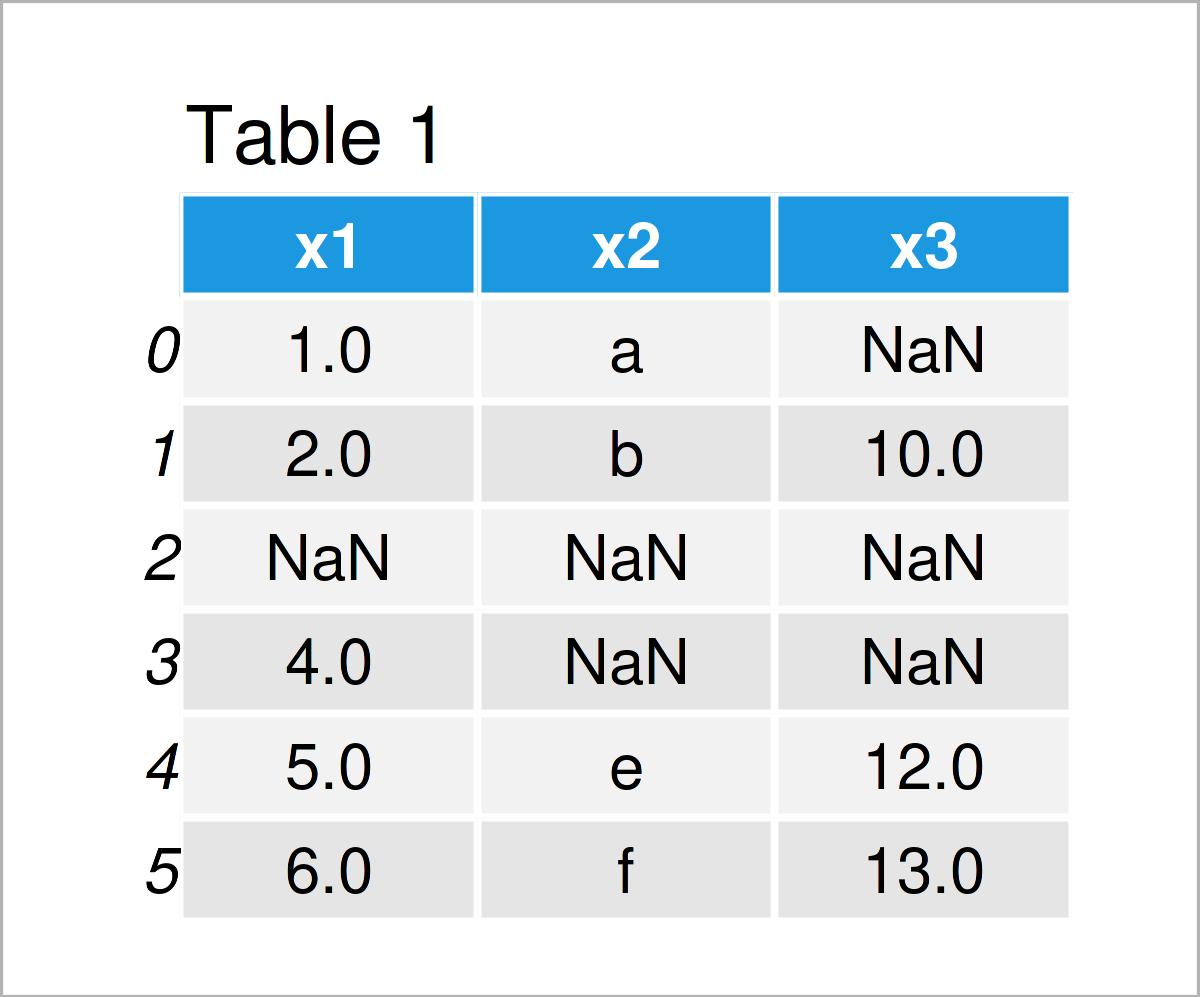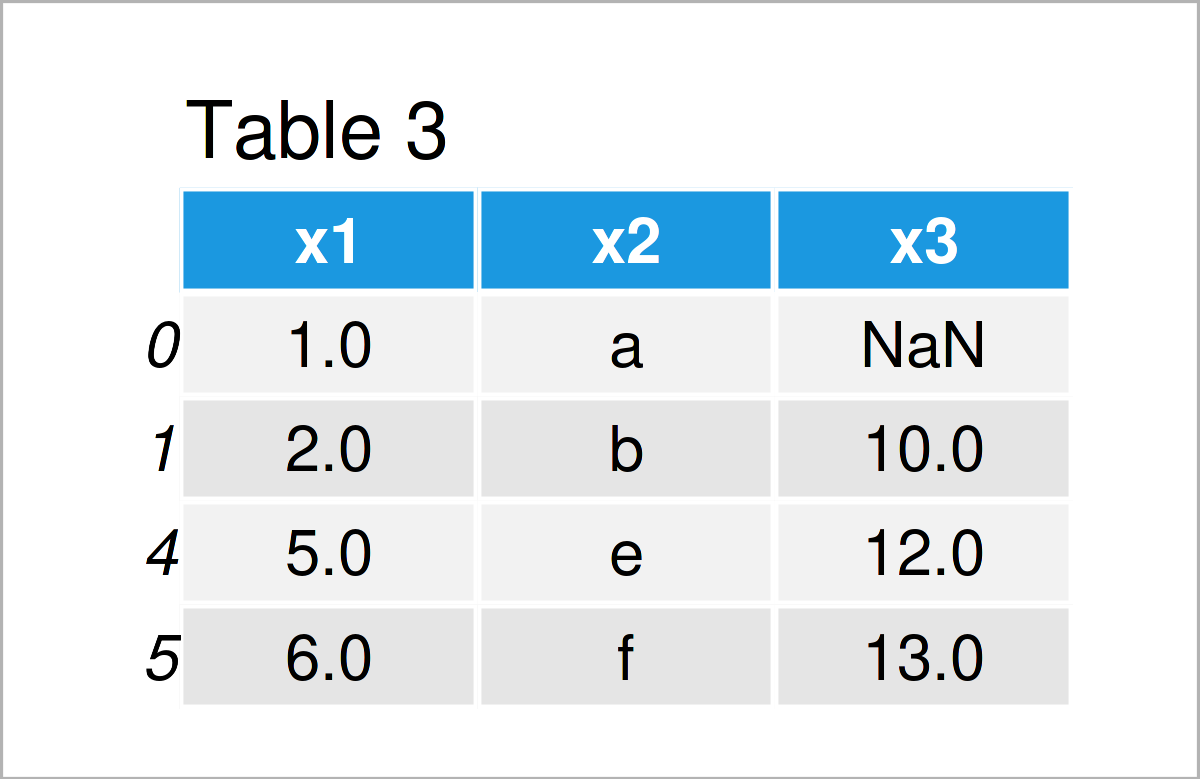# Remove Rows with NaN from pandas DataFrame in Python (4 Examples)

This article demonstrates how to drop rows containing NaN values in a pandas DataFrame in the Python programming language.

Let’s get started:

## Exemplifying Data & Add-On Packages

We first need to load the pandas library, if we want to use the functions that are contained in the library:

`import pandas as pd                             # Load pandas`

As next step, we’ll also have to create some exemplifying data.

```data = pd.DataFrame(                           # Create DataFrame with NaN values
{"x1":[1, 2, float("NaN"), 4, 5, 6],
"x2":["a", "b", float("NaN"), float("NaN"), "e", "f"],
"x3":[float("NaN"), 10, float("NaN"), float("NaN"), 12, 13]})
print(data)                                    # Print DataFrame with NaN values```Table 1 shows our example DataFrame. As you can see, it contains six rows and three columns. Multiple cells of our DataFrame contain NaN values (i.e. missing data).

In the following examples, I’ll explain how to remove some or all rows with NaN values.

## Example 1: Drop Rows of pandas DataFrame that Contain One or More Missing Values

The following syntax explains how to delete all rows with at least one missing value using the dropna() function.

Have a look at the following Python code and its output:

```data1 = data.dropna()                          # Apply dropna() function
print(data1)                                   # Print updated DataFrame```As shown in Table 2, the previous code has created a new pandas DataFrame, where all rows with one or multiple NaN values have been deleted.

## Example 2: Drop Rows of pandas DataFrame that Contain a Missing Value in a Specific Column

In Example 2, I’ll illustrate how to get rid of rows that contain a missing value in one particular variable of our DataFrame.

To make this work, we can use the subset argument of the dropna function:

```data2a = data.dropna(subset = ["x2"])          # Apply dropna() function
print(data2a)                                  # Print updated DataFrame```As shown in Table 3, we have created another pandas DataFrame subset. However, this time we have dropped only those rows where the column x2 contained a missing value.

Alternatively to the dropna function, we can also use the notna function…

```data2b = data[data["x2"].notna()]              # Apply notna() function
print(data2b)                                  # Print updated DataFrame```

…or the notnull function:

```data2c = data[pd.notnull(data["x2"])]          # Apply notnull() function
print(data2c)                                  # Print updated DataFrame```

All the previous Python codes lead to the same output DataFrame.

## Example 3: Drop Rows of pandas DataFrame that Contain Missing Values in All Columns

In Example 3, I’ll demonstrate how to drop only those rows of a pandas DataFrame where all variables of the DataFrame are not available.

For this, we have to specify the how argument of the dropna function to be equal to “all”.

```data3a = data.dropna(how = "all")              # Apply dropna() function
print(data3a)                                  # Print updated DataFrame```Table 4 shows the output of the previous Python code – A pandas DataFrame without all-NaN rows.

If we want to remove rows with only NaN values, we may also use notna function…

```data3b = data[data.notna().any(axis = 1)]      # Apply notna() function
print(data3b)                                  # Print updated DataFrame```

…or the notnull function:

```data3c = data[data.notnull().any(axis = 1)]    # Apply notnull() function
print(data3c)                                  # Print updated DataFrame```

## Example 4: Drop Rows of pandas DataFrame that Contain X or More Missing Values

This example demonstrates how to remove rows from a data set that contain a certain amount of missing values.

In the following example code, all rows with 2 or more NaN values are dropped:

```data4 = data.dropna(thresh = 2)                # Apply dropna() function
print(data4)                                   # Print updated DataFrame```In Table 5 you can see that we have constructed a new pandas DataFrame, in which we have retained only rows with less than 2 NaN values.

## Video & Further Resources on the Topic

Would you like to know more about removing rows with NaN values from pandas DataFrame? Then I can recommend having a look at the following video on my YouTube channel. In the video, I show the Python programming code of this article and give some explanations:

Please accept YouTube cookies to play this video. By accepting you will be accessing content from YouTube, a service provided by an external third party.If you accept this notice, your choice will be saved and the page will refresh.

Do you need more info on how to handle missing values in pandas DataFrames? Then you could have a look at the following video tutorial on the Data School YouTube channel:

Please accept YouTube cookies to play this video. By accepting you will be accessing content from YouTube, a service provided by an external third party.If you accept this notice, your choice will be saved and the page will refresh.

Furthermore, you might read some of the related articles on my website. You can find some posts below:

In summary: This article has demonstrated how to delete rows with one or more NaN values in a pandas DataFrame in the Python programming language. In case you have further questions, please let me know in the comments section. Besides that, don’t forget to subscribe to my email newsletter for updates on the newest articles.

Subscribe to the Statistics Globe Newsletter